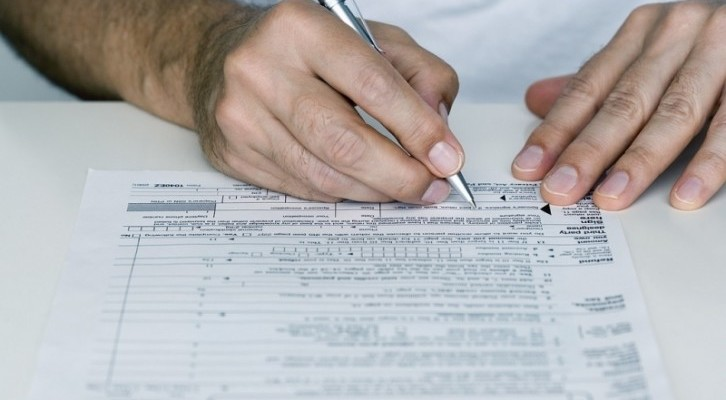Recently, we had posted two very interesting questions on prime numbers. Here are the solutions along with an interesting cross-section of the responses we got. Many thanks to Pratyush, Vamsigupta, Vishnu Sai, Sashank, Akarsh, Joel, Akanksa and a few others for contributing solutions.

Question 1: We want the smallest 5-digit snowball prime.

Solution 1: A snowball prime is also known as a right truncatable prime, which essentially means that if one starts removing the digits of the number from right, the number would still be a prime. The smallest 5-digit snowball prime is 23333. If you remove the right most digit, we are left with 2333 which also happens to be a prime. Same goes for 233, 23 and 2.

Solution 2: So I started off with 2(a prime number) and then to make it to 5 digit number first I made a 2-digit prime number i.e,I just put another 2 which became 22(since not a prime number) I went for 23 which is nice prime number.

Then further for 3-digit number since 232 didn’t satisfy I went on to 233 which is also a prime number.

Then for 4-digit number I directly put a 3 to make it 2333 and later a put another 3 which is 23333 and at last it is the smallest 5-digit prime number(snowball prime).  🙂

Question 2: There are 2-digit prime numbers ‘ab’ such that the prime number is the (a*b)th prime number. For instance, 17 is the 7th prime number and 73 is the 21st prime number. This is why some folks call 73 as the best number in the world, but that’s a discussion for another day. There is no three digit number ‘abc’ that is (a*b*c)th prime. However, there is/are perhaps one(or more)  3-digit number(s) ‘abc’ that is the (a*b*c)th odd prime. Give all possible such 3-digit prime numbers and pat yourself on the back.

Solution 1: Boy, this one took some time. The only way to approach this would be using a brute force method (Do tell me if there is another way). The only even prime number is 2. If a number is Nth prime, then it is (N-1)th odd prime. Looking for prime numbers between 100 and 999, we see that 829 is the 145th prime and 144th odd prime. Multiplying 8 by 2 by 9 gives 144. Hence, the answer is 829. There are no other prime numbers which satisfy this criteria in the aforementioned range.

Solution 2: For this question I simply generated all the prime numbers till 1000 using C language and then multiplied its digits and labeled its serial number (starting from 1 i.e with 3,first odd prime) and then if the condition is satisfied it’ll just print “yes”.

And then I found that there is only one such 3 digit number and that is 829(144th odd prime number).

Am thrilled that someone wrote a C program to answer one of our questions

Solution 3: This is my favorite solution. Carries rigor and intuition both

Method

0 can never be a prime number so checking for all those primes with zeroes would not add to the result.

Each number from 100-146(excluding 139 whose product is 27 but is the 33 odd prime) are not possible as there are 24 single and double  digit primes and the maximum product in these numbers is 24

Primes between 147 and 200 can be considered and range from 34 th odd  prime number to 45 the odd prime.

Another important point to note is that the numbers cannot have 2 1s as digits as it would lead to only a single digit product.It cannot have 2 2s as the maximum product is 36 and prime  numbers from 100 -200 are numbered well beyond that range.

In other words any combination of digits that yield a single digit product and double digit product less than 25 are not considered.

Considering the factors above we can eliminate a lot  of brute force checks.

Number of primes

Below 200- 46

Below 300 61

Below 400 77

Below 500  94

Belo 600 108

700 124

800 138

900 153

1000 167

First digit 1– product ranging between 31 to 46(considering few factors mentioned above)

Number product position

149 product 36 position 34

157(product 35 position 36

167 38th but product 42

179 63 is huge jump

197 and 199 also do not fall

So no prime of the desired requirement below 200

Between 200 to 300 we only need to look at even oddth primes as 2 would be one of the factors and must range between 47 and 61

48

50

52

54

56

58

60

48=2*24 283 is 60th prime

50 2*25 5*5 255 not prime

52 2*26 2*13*2 13 2 digit prime not possible

54 2*27 2*9*3 or 2*3*9

239 is 51st and 293 61 st so not possible

56 2*4*7  not prime

58 2*29 not possible

60 not possible

Therefore no luck  between 200-300

61-74 multiples of 3

63

Possibility

373  73 Rd

337 67th

66

3*11*2 not possible as 11 is 2 digit prime number

69 3*23 not possible as 23 is 2 digit prime

72 3*8*3 76th prime

With the similar format ,hence forth,

400-500

80 84 88 92

80 4*4*5 not prime

84 4*7*3 or 4*3*7not primes

88 4*11*2 not possible

92 4*23 not possible

500-600

95 100 105

95 5*19 not possible

100 5*5*4 not prime

105 5*5*7 101 the prime

600-700

108 114 120

108 6*2*9 not prime

114 6*19 not possible

120 6*4*5 not prime

700-800

126 7*2*9 not prime

133 7*19 not possible

800-900

144 8*2*9 prime and 144th odd prime so 829 is one such prime

152 8*19 not possible

900-1000

153 9*17 not possible

162 9*2*9 157th odd prime

So only 829 is one such prime which is 144th odd prime which is 8*2*9.